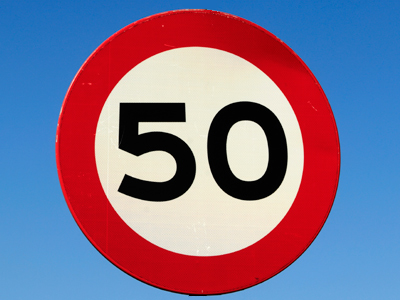All of the numbers in the 5 times table end with either 5 or 0.

# 5 Times Table

In KS1 Maths and Numeracy children will be exposed to the times tables as early as possible in Year 1. The 1, 2, 5 and 10 times tables are taught first before the 3, 4, 6, 7, 8 and 9 times tables follow. By the end of Year 2 children are often fluent in their times tables up to 10.

This quiz will test children's familiarity with their five times table, which will help them in studying maths in Years 1 and 2.

Do you know your five times table? Remember that 5 times a number is the same as half of ten times a number.

1.
5 x 2 =
7
20
10
15
To find 2 times a number you must double it
2.
5 x 5 =
10
15
25
30
5 x 5 is the same as 5 + 5 + 5 + 5 + 5
3.
5 x 7 =
35
34
36
32
All numbers in the 5 times table end with either 5 or 0
4.
5 x 4 =
30
25
16
20
5.
6 x 5 =
35
30
40
25
6.
9 x 5 =
15
50
40
45
7.
5 x 10 =
15
40
60
50
Remember, to find 10 times a number just add a 0 to the end of it
8.
8 x 5 =
40
35
45
60
One way to find 5 times a number is to half it and then times by 10.
Half of 8 is 4, and 4 x 10 = 40, so 8 x 5 = 40
9.
0 x 5 =
0
5
1
10
10.
1 x 5 =
1
5
10
6